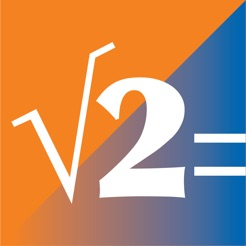iPhone Screenshots

•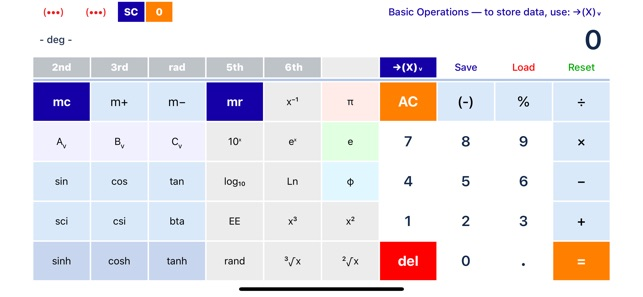•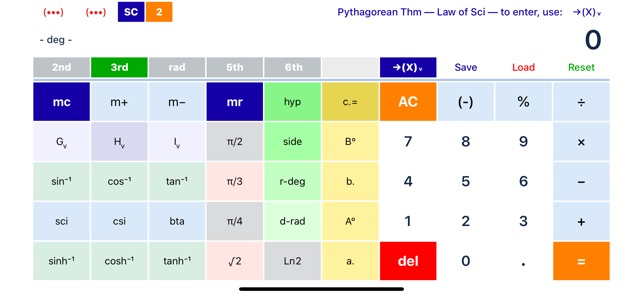•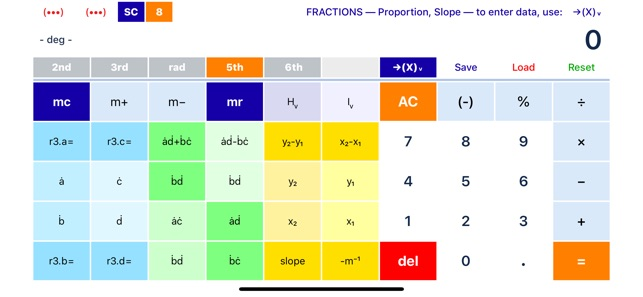•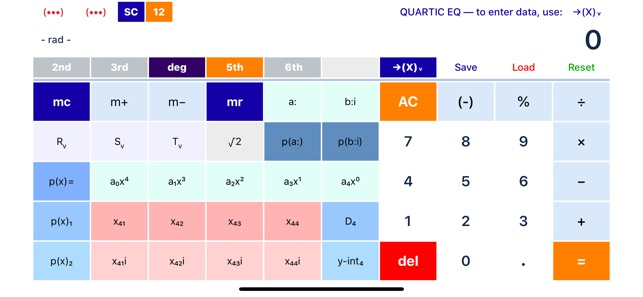•Description

"SciPro Math" is an extraordinary, polymorphic, scientific calculator, designed for portable devices, that contains hundreds of mathematical operations and has a great capability to store data. It can be used for doing unit conversions, algebra, geometry, trigonometry, pre-calculus, linear algebra, probability, and finance. It has been designed for both students and professionals.

FOR TUTORIALS, GO TO:

BENEFITS:
Introducing SciPro Math, the first Polymorphic Calculator. With the help of function keys to navigate, users discover that the App contains 16 different screens filled with all kinds of exotic mathematical operations. It works like magic.

Using storage variables to hold and store the values of the coefficients, you are now able to enter entire linear, quadratic, cubic, and even quartic equations right on the application itself, and save, solve, and evaluate them, live.

There is no need to write that much. Just solve and store the solutions right on the calculator. It has a great storage capability available for you to use.

Use the calculator for doing unit conversions within a particular system of measurements, but also for performing unit conversions across different systems, namely: the imperial and metric.

Instead of doing complex calculations by hand, like evaluating equations, avoid making silly mistakes, let "SciPro Math" do them for you.

TOPICS:
"SciPro Math" is the perfect companion for your mathematics class. Consider it for the following topics and subjects:
• Arithmetic
• Conversions
• Algebra
• Linear Algebra
• Geometry
•  Trigonometry
•  Probability
• Precalculus
• Finance

FEATURES:
•  Screen identification numbers: 0 – 24
•  A new navigation system that uses the screen numbers: 0 – 24
•  Screen numbers, titles, descriptions, and functional instructions, as follows:
•  0 – Basic Operations — to store data, use: ->(X)v
•  1 – Basic Operations — to store data, use: ->(X)v
•  2 – Pythagorean Thm — Law of Sci — to enter, use: ->(X)v
•  3 – PROBABILITY — Comb, Perm — TEMPERATURE
•  4 – Mixed Conversion — to store data, use: ->(X)v
•  5 – Measure Conversion — to store data, use: ->(X)v
•  6 – Volume Conversion — to store data, use: ->(X)v
•  7 – Weight Conversion — to store data, use: ->(X)v
•  8 – FRACTIONS — Proportion, Slope — to enter data, use: ->(X)v
•  9 – LINEAR EQ — Slope — to enter data, use: ->(X)v
•  10 – QUADRATIC EQ — to enter data, use: ->(X)v
•  11 – CUBIC EQ — to enter data, use: ->(X)v
•  12 – QUARTIC EQ — to enter data, use: ->(X)v
•  13 – FINANCE — percent, interest — to enter data, use: ->(X)v
•  14 – FINANCE — to enter data, use: ->(X)v
•  15 – LINEAR ALGEBRA — System of 2 EQ — to enter, use: ->(X)v
•  16 – LINEAR ALGEBRA — System of 3 EQ — to enter, use: ->(X)v
•  17 – LINEAR ALGEBRA — Solutions for 3 EQ — by Gauss-Jordan
•  18 – LINEAR ALGEBRA — System of 4 EQ — to enter, use: ->(X)v
•  19 – LINEAR ALGEBRA — Solutions for 4 EQ — by Gauss-Jordan
•  21 – GEOMETRY — Area, Volume — to enter data: ->(X)v
•  22 – GEOMETRY — Pyramid, Cylinder, Cone — Sector, Segment
•  23 – GEOMETRY — Platonic Solids — Hollow Cylinder (Tube)
•  24 – GEOMETRY — Platonic Solids — to enter data: ->(X)v
•  25 – STORAGE SPACE — to store data, use: ->(X)v

MORE FEATURES:
• Extensive button-click descriptions for “How To Use”
• The Navigation System now contain the functions: 2nd, 3rd, rad, 5th, 6th
• The Navigation System manages 24 Screens, and it can manage up to 32, leaving a lot of room for future grows
• The number of screens increased from 16 to now 24
• It has 648 mathematical commands
• A color management system
• The number of managed colors is now 140
•  Memory variables: Av to Zv
•  A Value Transfer Function “->(X)v” that is used to store numbers in the application
•  Save program function
• Color scheme switch
•  Reset function

What’s New

Version 4.0

Version 4.0

We thank you for choosing “SciPro Math” as your primary mathematical tool. To make this App better, we bring updates to the AppStore as often as necessary to improve its performance and add functionality.

• This new version includes:
• Better stability look and feel
• Reengineering of all the color schemes
• The number of managed colors is now 140
• Extensive button-click descriptions for “How To Use”
• The Navigation System now contains the functions: 2nd, 3rd, rad, 5th, 6th
• The Navigation System manages 24 Screens, and it can manage up to 32, leaving a lot of room for future grows
• It has 648 mathematical commands
• Solving Fractions: Addition, subtraction, multiplication, and Division: for a/b, c/d
• Solving Fractions: Slope calculation, indication the numerator and the denominator
• Solving proportions: The Rules of Three for: a/b = c/d
• Linear Algebra: Solving systems of 2, 3, and 4 linear equations by Gauss-Jordan Elimination
• Solving systems of more equations than unknowns and more unknowns than equation of linear algebra by Gauss-Jordan Elimination
• It evaluates the solutions of system of linear equations on the original equations to confirm the answers given
• Finance, it now includes Straight-Line depreciation and declining balance depreciation
• Quadric, Cubic, and Quartic Equations are presented in separate screens, they now all evaluate imaginary numbers
• Quadric, Cubic, and Quartic Equations now all evaluate the first and second derivative of the function and a given point to determine the critical points of a function, relative maxima, relative minima, inflection points, and concavity.
• Circle and Spheres: it now calculates sectors segments, and caps: Area, Volume, Surface Area, Radius of base circle, lateral surface area, and center of gravity.
• Hollow Cylinder: Volume, Surface Area, lateral surface area, and center of gravity.
• Tetrahedron, Cube, Octahedron, Dodecahedron, Icosahedron: Volume, Surface Area, number of sides, number of edges, number of vertices, Circum-Radius, and In-Radius.

As new features become available, we will highlight them here for you. “SciPro Math” is a truly worthwhile, modern mathematical tool.

Ratings and Reviews

5.0 out of 5
53 Ratings

53 Ratings

Matt the MathMan ,

WORTH EVERY PENNY!!!

I have come across many different calculators over the years, but had never heard of a polymorphic calculator like SciPro Math. I got the App out of curiosity and discovered what it really means. It is a way to fit a lot of operations into the keyboard, then uses functions to change the look of the keys. This is ingenious. I have not seen a calculator that does anything close to what SciPro Math does. I lost count of the amount of operations that it contains. In all honesty, this is the best calculator I have ever seen. I wholeheartedly recommend it to everyone out there. This is one investment for a lifetime. The best thing is that you can have all this computing power right in your phone.  Best of luck to you!

Developer Response , 03/29/2019

🌈▪️Yes, thanks. Please spread the word ! 📱Enjoy the new version: 🔶
You may find tutorial videos on how to use at "www.scipromath.com" or search for “SciPro Math” on YouTube: 🔎. Besides simple math, “SciPro Math” has a lot of advanced features. And, after watching one video, learning how to use will become common sense.
💬 Remember, in order to enter values into the calculator — that is: values from equations or to be stored — please use the Value Transfer Function: ->(X). This key is located on top of the AC key.▪️

Americanito ,

A POLYMORPHIC CALCULATOR !!! 👀

🙂 SciPro Math surpasses all my expectations for a calculator. It has lots of functionalities and documentation to make it easy for me to find what I need. It offers 2 navigation system: the first one uses function keys; the second uses screen numbers. They come in very handy. It is simply amazing how the developers were able to package over 450 operations in such a limited amount of space and still maintain a high standard of comfort and aesthetics in the process. This application is truly worth it. 🙏

Developer Response , 02/26/2018

Super! Thanks for your kind words of recognition for the work that has gone into this project...

rellz9000 ,

Game Changer!!!!

Been struggling a little bit with math and stubble upon this app and I have to say WOWWWW what a GAME CHANGER! I’m not big on giving reviews but this has all you need in-order to be great with mathematical problems and work. Truly exited and happy to have this app. My family uses it and I at work!

Developer Response , 01/15/2019

💢 ▪️Yes, thanks. Please spread the word ! 📱Enjoy the new version: 🔵
You may find tutorial videos on how to use at "www.scipromath.com" or search for “SciPro Math” on YouTube: 🔎. Besides simple math, “SciPro Math” has a lot of advanced features:. And, after watching one video, learning how to use will become common sense. Remember, to enter values into the calculator, from equations or to be stored, please use the value transfer function: ->(X). This key is located on top of the AC key.▪️

Information

Seller
Roberto Antonio Campusano Acosta
Size
18.2 MB
Category
Utilities
Compatibility

Requires iOS 9.3 or later. Compatible with iPhone, iPad, and iPod touch.

Languages

English

Age Rating
Rated 4+
•